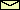The Equations of Motion

How can we gain a understanding of whats happening in the lab? In physics the traditional way of thinking is to find the law behind the phenomena. For a mechanical system like the pendulum this means to find all forces governing the motion. That is, to find the equation of motion. First, the equation of motion for the undamped and undriven pendulum will be derived. This is easy. Maybe you know it already from a freshman course in physics. A bit more complicated is the derivation of the equation of motion for various damped and driven pendula. In physical textbooks quite often only the linearized equation of motions are discussed. Yet, most of the phenomena you can observe in the Pendulum Lab are caused by the nonlinearity in the equation of motion. Nonlinear dynamics is therefore the main topic of this mini lecture.

© 1998 Franz-Josef Elmer,Franz-Josef doht Elmer aht unibas doht ch, last modified Sunday, July 19, 1998.